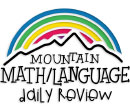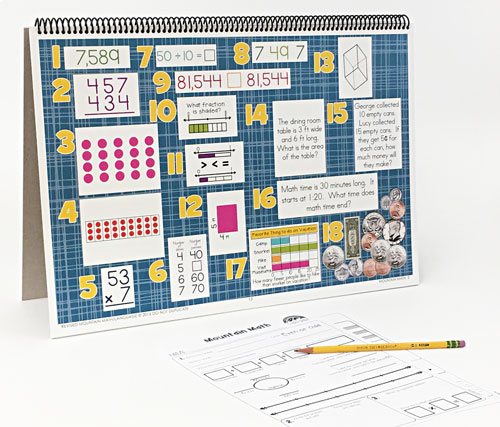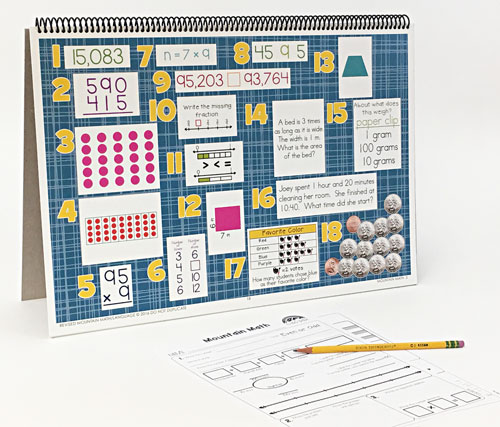WHAT YOU GET:
• 32 WEEKS OF DAILY REVIEW
• INSTRUCTIONS
• ONE MASTER STUDENT RESPONSE SHEET
• HEAVY DUTY STAND
• KNOWLEDGEABLE STAFF FOR ANY ASSISTANCE

This 3rd GRADE MOUNTAIN MATH CENTER EDITION is the DAILY REVIEW your students need to MASTER the concepts you have taught.

Research has proven that LONG-TERM MASTERY comes from SPIRAL REVIEW, also called DISTRIBUTED PRACTICE AND SPACED REPETITION.

The CENTER EDITION is designed for small group review, such as rotations, scoots, centers, or math-around-the-room. It is a smaller version (13"x19") of our Bulletin Board Kit. It can easily be set up and taken down as needed.
1. Compose and decompose numbers up to 100,000 as a sum of so many ten thousands, so many thousands, so many hundreds, so many tens, and so many ones using objects, pictorial models, and numbers, including expanded form as appropriate.
Describe the mathematical relationships found in the base-10 place value system through the hundred thousands place.
Represent a number on a number line as being between two consecutive multiples of 10; 100; 1,000; or 10,000 and use words to describe relative size of numbers in order to round whole numbers.
Round to the nearest 10 or 100 or use compatible numbers to estimate solutions to addition and subtraction problems.
Determine if a number is even or odd using divisibility rules.
2. Solve with fluency one-step and two-step problems involving addition and subtraction within 1,000 using strategies based on place value, properties of operations, and the relationship between addition and subtraction.
Represent one- and two-step problems involving addition and subtraction of whole numbers to 1,000 using pictorial models, number lines, and equations.
3. Determine the total number of objects when equally-sized groups of objects are combined or arranged in arrays up to 10 by 10.
Represent multiplication facts by using a variety of approaches such as repeated addition, equal-sized groups, arrays, area models, equal jumps on a number line, and skip counting.
Solve one-step and two-step problems involving multiplication and division within 100 using strategies based on objects; pictorial models, including arrays, area models, and equal groups; properties of operations; or recall of facts.
4. Determine the number of objects in each group when a set of objects is partitioned into equal shares or a set of objects is shared equally.
Determine if a number is even or odd using divisibility rules.
Determine a quotient using the relationship between multiplication and division.
Represent and solve one- and two-step multiplication and division problems within 100 using arrays, strip diagrams, and equations.
Solve one-step and two-step problems involving multiplication and division within 100 using strategies based on objects; pictorial models, including arrays, area models, and equal groups; properties of operations; or recall of facts.
5. Use strategies and algorithms, including the standard algorithm, to multiply a two-digit number by a one-digit number. Strategies may include mental math, partial products, and the commutative, associative, and distributive properties.
6. Represent real-world relationships using number pairs in a table and verbal descriptions.
7. Recall facts to multiply up to 10 by 10 with automaticity and recall the corresponding division facts.
Use strategies and algorithms, including the standard algorithm, to multiply a two-digit number by a one-digit number. Strategies may include mental math, partial products, and the commutative, associative, and distributive properties.
Determine the unknown whole number in a multiplication or division equation relating three whole numbers when the unknown is either a missing factor or product.
8. Recall facts to multiply up to 10 by 10 with automaticity and recall the corresponding division facts.
Determine a quotient using the relationship between multiplication and division.
9. Compare and order whole numbers up to 100,000 and represent comparisons using the symbols >, <, or =.
10. Represent fractions greater than zero and less than or equal to one with denominators of 2, 3, 4, 6, and 8 using concrete objects and pictorial models, including strip diagrams and number lines.
Determine the corresponding fraction greater than zero and less than or equal to one with denominators of 2, 3, 4, 6, and 8 given a specified point on a number line.
Explain that the unit fraction 1/b represents the quantity formed by one part of a whole that has been partitioned into b equal parts where b is a non-zero whole number.
Compose and decompose a fraction a/b with a numerator greater than zero and less than or equal to b as a sum of parts 1/b.
Solve problems involving partitioning an object or a set of objects among two or more recipients using pictorial representations of fractions with denominators of 2, 3, 4, 6, and 8.
Decompose two congruent two-dimensional figures into parts with equal areas and express the area of each part as a unit fraction of the whole and recognize that equal shares of identical wholes need not have the same shape.
11. Represent fractions of halves, fourths, and eighths as distances from zero on a number line.
Represent equivalent fractions with denominators of 2, 3, 4, 6, and 8 using a variety of objects and pictorial models, including number lines.
Explain that two fractions are equivalent if and only if they are both represented by the same point on the number line or represent the same portion of a same size whole for an area model.
Compare two fractions having the same numerator or denominator in problems by reasoning about their sizes and justifying the conclusion using symbols, words, objects, and pictorial models.
Decompose two congruent two-dimensional figures into parts with equal areas and express the area of each part as a unit fraction of the whole and recognize that equal shares of identical wholes need not have the same shape.
Represent fractions of halves, fourths, and eighths as distances from zero on a number line.
12. Determine the area of rectangles with whole number side lengths in problems using multiplication related to the number of rows times the number of unit squares in each row.
Decompose composite figures formed by rectangles into non-overlapping rectangles to determine the area of the original figure using the additive property of area.
13. Classify and sort two- and three-dimensional figures, including cones, cylinders, spheres, triangular and rectangular prisms, and cubes, based on attributes using formal geometric language.
Use attributes to recognize rhombuses, parallelograms, trapezoids, rectangles, and squares as examples of quadrilaterals and draw examples of quadrilaterals that do not belong to any of these subcategories.
14. Solve one-step and two-step problems involving multiplication and division within 100 using strategies based on objects; pictorial models, including arrays, area models, and equal groups; properties of operations; or recall of facts.
Determine the perimeter of a polygon or a missing length when given perimeter and remaining side lengths in problems.
15. Solve one-step and two-step problems involving multiplication and division within 100 using strategies based on objects; pictorial models, including arrays, area models, and equal groups; properties of operations; or recall of facts.
Represent one- and two-step problems involving addition and subtraction of whole numbers to 1,000 using pictorial models, number lines, and equations.
Represent and solve one- and two-step multiplication and division problems within 100 using arrays, strip diagrams, and equations.
Determine when it is appropriate to use measurements of liquid volume (capacity) or weight.
Determine liquid volume (capacity) or weight using appropriate units and tools.
16. Determine the solutions to problems involving addition and subtraction of time intervals in minutes using pictorial models or tools such as a 15-minute event plus a 30-minute event equals 45 minutes.
17. Summarize a data set with multiple categories using a frequency table, dot plot, pictograph, or bar graph with scaled intervals.
Solve one- and two-step problems using categorical data represented with a frequency table, dot plot, pictograph, or bar graph with scaled intervals.
18. Determine the value of a collection of coins and bills.
TEXAS ESSENTIAL KNOWLEDGE AND SKILLS CORRELATION
1. 3.2ABC, 3.4BJ
2. 3.4A, 3.5A
3. 3.4DE, 3.5B
4. 3.4HIJ, 3.5B
5. 3.4G
6. 3.5E
7. 3.4FG, 3.5D
8. 3.4FJ
9. 3.2D
10. 3.3ABCDE, 3.6E, 3.7A
11. 3.3FGH, 3.6E, 3.7A
12. 3.6CD
13. 3.6AB
14. 3.6C, 3.7B
15. 3.4K, 3.5AB, 3.7DE
16. 3.7C
17. 3.8AB
18. 3.4C
ONLINE REVIEW VS.
CENTER EDITION VS.
BULLETIN BOARD KIT
ONLINE REVIEW
Is projected onto an interactive white board, creating a virtual board. It can be viewed by the entire classroom. Answers can be displayed onto the board at the click of a button. Up to seven questions can be isolated and magnified.

CENTER EDITION
Is organized on a 13"x19" flip chart. It can be viewed by 5-6 students at a time. It was designed for small group review, such as rotations, scoots, centers, or math-around-the-classroom. It has an answer key, heavy-duty stand, and high-gauged coil.

BULLETIN BOARD KIT
It is designed for total classroom view. It hangs on bulletin boards, walls, white boards, pocket charts, and science display boards. Once hung, it can remain displayed for the entire year. The cards can be interchanged, creating flexible and adjustable DAILY REVIEW.
\$99.95 QTY: